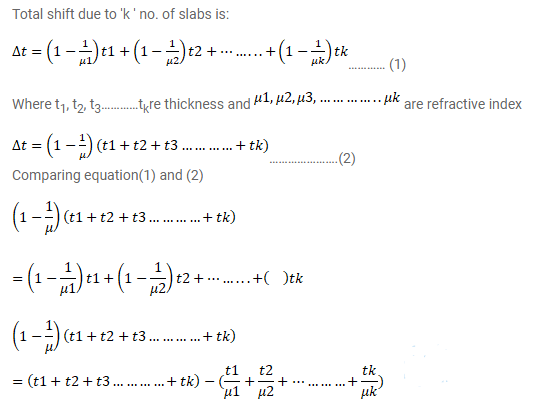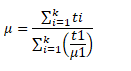# k transparent slabs are arranged one over another.Question:

$k$ transparent slabs are arranged one over another. The refractive index of the slabs are $\mu_{1}, \mu_{2}, \mu_{3}, \ldots \ldots \ldots \ldots \ldots \mu_{\mathrm{k}}$ and the thickness are $t_{1}, t_{2}, t_{3} \ldots \ldots \ldots \ldots t_{k}$. An object is seen through this combination with nearly perpendicular light. Find the equivalent refractive index of the system which will allow the image to be formed at the same place.

Solution: